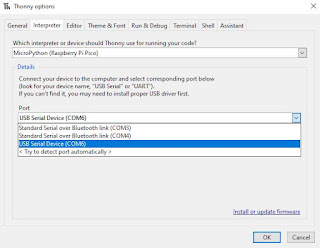## Shop# 74ls181 ALU 4-Bit Logic Unit TTL

What does a 4-Bit ALU do?

Written by: Larsha Johnson
6/16/2015

The 74181 is still used today in retro hacker projects. Here's ow it works and why it's so strange. Is it an all in one logic chip? Well, yes. Since it provides 16 Arithmetic Operations Add, Subtract, Compare, Double, Plus Twelve Other Arithmetic Operations as well as provides all 16 Logic Operations of Two Variables Exclusive — OR( XOR), Compare, AND, NAND, OR, NOR, Plus Ten other Logic Operations!

Why was it designed? To make computing faster in comparison with standard logic gates that was what available at the time. In March 1970, Texas Instruments introduced the 74181 Arithmetic / Logic Unit (ALU) chip, which put a full 4-bit ALU on one fast TTL chip. This chip provided 32 arithmetic and logic functions, as well as carry lookahead for high performance.

The SN54/74LS181 is a 4-bit Arithmetic Logic Unit (ALU) which can perform all the possible 16 logic, operations on two variables and a variety of arithmetic operations.

The 74181 ALU (arithmetic/logic unit) chip powered many of the minicomputers of the 1970s: it provided fast 4-bit arithmetic and logic functions, and could be combined to handle larger words, making it a key part of many CPUs.

The 74181 is a 4-bit slice arithmetic logic unit (ALU), implemented as a 7400 series TTL integrated circuit. The first complete ALU on a single chip, it was used as the arithmetic/logic core in the CPUs of many historically significant minicomputers and other devices.

The 74181 represents an evolutionary step between the CPUs of the 1960s, which were constructed using discrete logic gates, and today's single-chip microprocessor CPUs. Although no longer used in commercial products, the 74181 is still referenced in computer organization textbooks and technical papers. It is also sometimes used in 'hands-on' college courses, to train future computer architects.

• Provides 16 Arithmetic Operations Add, Subtract, Compare, Double,
Plus Twelve Other Arithmetic Operations
Provides all 16 Logic Operations of Two Variables Exclusive — OR,
Compare, AND, NAND, OR, NOR, Plus Ten other Logic Operations
• Full Lookahead for High Speed Arithmetic Operation on Long Words
• Input Clamp Diodes

FUNCTIONAL DESCRIPTION

The SN54/74LS181 is a 4-bit high speed parallel Arithmetic Logic Unit (ALU). Controlled by the four Function Select Inputs (S0 . . . S3) and the Mode Control Input (M), it can perform all the 16 possible logic operations or 16 different arithmetic operations on active HIGH or active LOW operands. The Function Table lists these operations.

The combinational logic circuitry of the 74181 integrated circuit

Reference articles